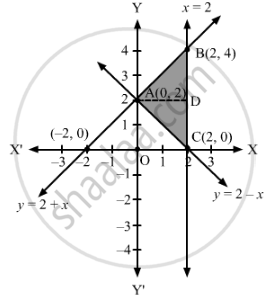# Using integration, find the area of the region bounded by the lines y = 2 + x, y = 2 – x and x = 2. - Mathematics

Using integration, find the area of the region bounded by the lines y = 2 + x, y = 2 – x and x = 2.

#### Solution

The equations of the given lines are

y = 2 + x    .....(1)

y = 2 – x    .....(2)

x = 2          .....(3)

Solving (1), (2) and (3) in pairs, we obtain the coordinates of the point of intersection as A(0, 2), B(2, 4) and C(2, 0).

The graphs of these lines are drawn as shown in the figure below.Here, the shaded region represents the area bounded by the given lines.

∴ Required area = Area of the region ABCA

= Area of region ACDA + Area of region ABDA

=∫_0^2xAC dy+∫_2^4xAB dy

=∫_0^2(2−y)dy+∫_2^4(y−2)dy

=(2y-y^2/2)_0^2+(Y^2/2-2y)_2^4

=[(42)(00)]+[(88)(24)]

=2+2

=4 square units

Thus, the area of the region bounded by the given lines is 4 square units.

Concept: Area of the Region Bounded by a Curve and a Line
Is there an error in this question or solution?